#### 期刊菜单

Research on Image Reversible Double Watermarking Algorithm in Ciphertext Domain Based on NTRU
DOI: 10.12677/SEA.2022.113053, PDF, HTML, XML, 下载: 82  浏览: 145  国家自然科学基金支持

Abstract: In this paper, the digital image and string information are encrypted based on NTRU cryptographic algorithm. Using the homomorphic characteristics of NTRU cryptographic algorithm, different users can embed the watermark information in the ciphertext domain twice in different stages; the receiver can recover the original work information and the watermark information embedded twice by processing the ciphertext image with NTRU decryption algorithm. Through comparison, it is found that the algorithm can completely restore the original work information and embedded watermark information, and can achieve the expected effect. At the same time, the algorithm is based on post quantum cryptography algorithm, which can resist the attack of quantum computer, and the embedding amount of watermark information is larger. It can embed watermark information of both sides, and the embedding and extraction are not disturbed and affected by each other.

1. 引言

2. NTRU算法及同态特性分析

2.1. NTRU算法

NTRU加密系统最早由三位数学家Jill Pipher，Jeffrey Hoffstein，Joseph Silverman提出 。这种加密系统的数学核心是多项式环截断环，加密系统的可靠性由格上最短向量问题和最近向量问题保证，这属于NP难(non-deterministic polynomial hard)问题。由于加密和解密过程中只运用到简单的模乘与加减运算，在相同安全性等级的前提下，NTRU比目前现有的公钥密码系统运算更快，效率更高，具有很广阔的应用前景。

2.1.1. NTRU密码体制及其基本运算操作

NTRU算法定义在多项式环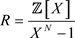上，R上的元素——多项式 $f\left(x\right)$ 也可以用向量表达： $f={\sum }_{i=0}^{N-1}{f}_{i}\cdot {x}^{i}=\left[{f}_{0},{f}_{1},\cdots ,{f}_{N-1}\right]$。对于环中的任意两个多项式 $f\left(x\right)$$g\left(x\right)$ 可以表示为以下的形式： $f={f}_{0}+{f}_{1}\cdot x+\cdots +{f}_{N-1}\cdot {x}^{N-1}$$g={g}_{0}+{g}_{1}\cdot x+\cdots +{g}_{N-1}\cdot {x}^{N-1}$。元素之间存在多种运算，包括：

$f\left(x\right)+g\left(x\right)={\sum }_{i=0}^{N-1}\left({f}_{i}+{g}_{i}\right)\cdot {x}^{i}$ (2-2)

$f\left(x\right)\ast g\left(x\right)={\sum }_{i=0}^{N-1}\left({\sum }_{i+j=k\left(\mathrm{mod}\text{ }N\right)}{f}_{i}\cdot {g}_{j}\right)\cdot {x}^{i}$ (2-3)

2.1.2. NTRU加解密参数

NTRU密码体制由3个正整数 $\left(N,p,q\right)$ 和4个整系数多项式集合 ${L}_{f},{L}_{g},{L}_{r}$${L}_{m}$ 共同决定。其中正整数p和q的选取满足 $\mathrm{gcd}\left(p,q\right)=1$ 且q远大于p。用“*”乘表示环R中的乘法，在整个密码系统中，一部分乘法将在模q下运算，另一部分将在模p下运算。多项式 ${L}_{f},{L}_{g},{L}_{r}$${L}_{m}$ 的选取应遵循以下原则：明文m所选取的集合 ${L}_{m}$ 是包括所有模p的多项式。这里为了方便讨论，假设p是奇数，于是有

${L}_{m}=\left\{m\in R:m\text{ }\text{\hspace{0.17em}}的系数位于-\frac{p-1}{2}和\frac{p-1}{2}之间\right\}$

$L\left({d}_{1},{d}_{2}\right)=\left\{F\in R:F\text{ }\text{ }中有\text{ }\text{ }{d}_{1}\text{ }\text{ }个系数等于\text{ }\text{ }1,\text{\hspace{0.17em}}{d}_{2}\text{ }\text{ }个系数等于-1,\text{\hspace{0.17em}}剩下的系数为\text{ }\text{ }0\right\}$

2.1.3. NTRU算法加密解密过程

2.1.4. NTRU安全等级

Silverman等人给出了NTRU不同的参数选取方案，以此来获得不同的安全等级。在NTRU公钥密码的原始方案中，N = 107规模的参数对应了中等安全性密码系统。表1是NTRU-1998参数集对应的安全性等级 。Table 1. Recommended parameters for different security levels of NTRU

2.2. NTRU同态性质

NTRU存在加法同态性：对于任意的两个明文多项式 ${m}_{1}$${m}_{2}$，选择两个随机的多项式 ${r}_{1}$${r}_{2}$，则经过加密之后对应生成的密文为 ${e}_{1}$${e}_{2}$，其中满足：

$P{T}^{*}={\sum }_{i=0}^{N-1}\left(p{{t}^{\prime }}_{i}+p{{t}^{″}}_{i}\right)\cdot {x}^{i}=\left[p{{t}^{\prime }}_{0}+p{{t}^{″}}_{0},p{{t}^{\prime }}_{1}+p{{t}^{″}}_{1},\cdots ,p{{t}^{\prime }}_{N-2}+p{{t}^{″}}_{N-2},p{{t}^{\prime }}_{N-1}+p{{t}^{″}}_{N-1}\right]$

3. 双水印算法设计

3.1. 算法流程

3.2. 信息编码与加密解密

3.2.1. 字符串信息编码

3.2.2. 图像信息编码

3.2.3. 加密明文多项式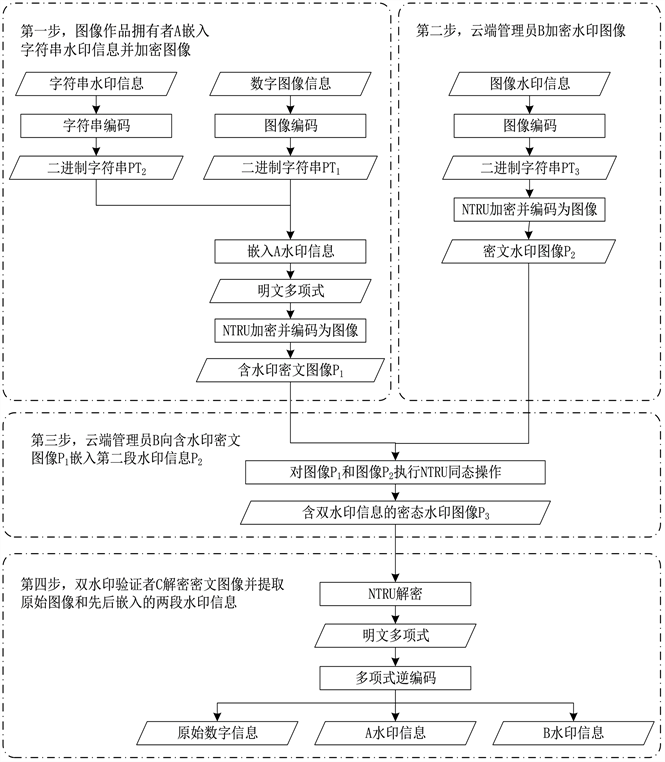Figure 1. Flowchart of double watermarking algorithm in ciphertext domain based on NTRU

3.2.4. 解密密文多项式

3.3. 具体过程

3.3.1. 图像作品拥有者A嵌入字符串水印信息并加密图像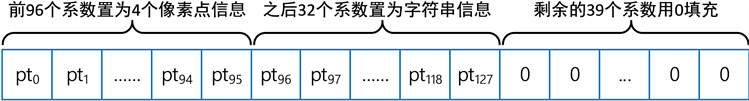Figure 2. Schematic diagram of encoding original image and string watermark information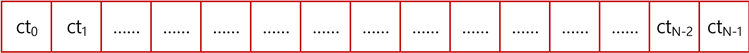Figure 3. Schematic diagram of ciphertext polynomial

3.3.2. 云端管理员B加密水印图像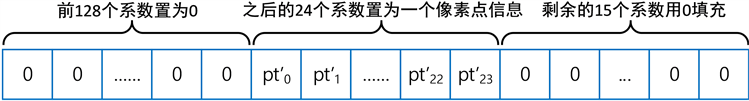Figure 4. Constructing plaintext polynomial of watermark image in cloud

3.3.3. 云端管理员B嵌入第二段水印信息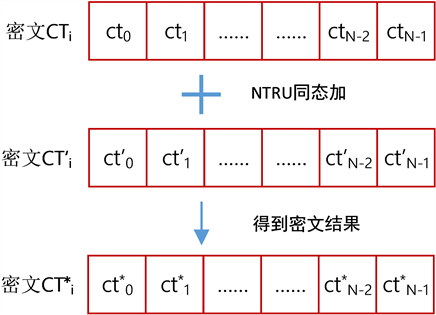3.3.4. 水印信息验证者C解密提取原始图像和双水印信息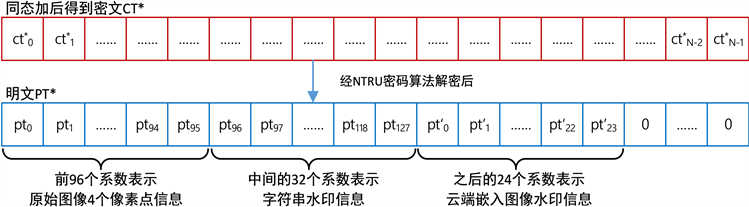Figure 6. The decryptor provides the private key for decryption

4. 实验与分析

4.1. 实验过程Figure 7. Original image informationFigure 8. Image watermark information(a)(b)(c)

Figure 9. (a) Ciphertext Image P1; Ciphertext Image P2; Ciphertext Image P3Figure 10. Histogram statistical results of watermark image containing both watermark informationFigure 11. Recover the original image information after decryptionFigure 12. Recover the image watermark information after decryption

4.2. 运行效率Table 2. Time cost of encrypting and decrypting 2048 polynomials under different security levels of NTRU

4.3. 安全性分析

5. 总结

  傅楚君, 兰胜坤. 基于DCT变换的数字水印算法[J]. 网络安全技术与应用, 2020(7): 49-51.  胡坤, 李聪, 胡建平, 王小超, 杜玲, 王红飞. 基于BEMD与DCT的彩色图像多重水印鲁棒算法[J/OL]. 北京航空航天大学学报, 1-16. https://doi.org/10.13700/j.bh.1001-5965.2021.0214  王东东, 王福明. 基于LSB数字水印算法的研究与实现[J]. 山西电子技术, 2014(5): 76-77.  Zhang, X. (2011) Reversible Data Hiding in Encrypted Image. IEEE Signal Processing Letters, 18, 255-258. https://doi.org/10.1109/LSP.2011.2114651  Chen, Y.C., Shiu, C.W. and Horng, G. (2014) Encrypted Signal-Based Reversible Data Hiding with Public Key Cryptosystem. Journal of Visual Communication and Image Representation, 25, 1164-1170. https://doi.org/10.1016/j.jvcir.2014.04.003  Ke, Y., Zhang, M. and Su, T. (2016) A Novel Multiple Bits Reversible Data Hiding in Encrypted Domain Based on R-LWE. Journal of Computer Research & Development, 53, 2307-2322.  Zhou, N., Zhang, M.Q., Zhou, H.N., et al. (2020) Reversible Data Hiding Algorithm in Encrypted Domain Based on NTRU. Science Technology and Engineering, 20, 13285-13294. (in Chinese)  Zhou, N., Zhang, M., Wang, H., et al. (2020) Separable Reversible Data Hiding Scheme in Homomorphic Encrypted Domain Based on NTRU. IEEE Access, 8, 81412-81424.  项世军, 罗欣荣, 石书协. 一种同态加密域图像可逆水印算法[J]. 计算机学报, 2016, 39(3): 571-581.  Paillier, P. (1999) Public-Key Cryptosystems Based on Discrete Logarithms Residues. Advances in Cryptology-Eu- rocrypt’99, International Conference on the Theory and Application of Cryptographic Techniques, Prague, Czech Republic, 2-6 May 1999, 223-238.  Brakerski, Z. and Vaikuntanathan, V. (2014) Efficient Fully Homomorphic Encryption from (Standard) Lwe. 2011 IEEE 52nd Annual Symposium on Foundations of Computer Science, pp. 97-106.  Gentry, C. (2009) Fully Homomorphic Encryption Using Ideal Lattices. STOC, 9, 169-178. https://doi.org/10.1145/1536414.1536440  Fan, J. and Vercauteren, F. (2012) Somewhat Practical Fully Homomorphic Encryption. Iacr Cryptology ePrint Archive, 2012, 144.  Hoffstein, J., Pipher, J. and Silverman, J.H. (1998) NTRU: A Ring-Based Public Key Cryptosystem. In: Buhler, J.P., (Ed.), Algorithmic Number Theory, Springer, Heidelberg. https://doi.org/10.1007/BFb0054868  Wu, H.T., Cheung, Y., Yang, Z., et al. (2019) A High-Capacity Reversible Data Hiding Method for Homomorphic Encrypted Images. Journal of Visual Communication and Image Representation, 62, 87-96. https://doi.org/10.1016/j.jvcir.2019.04.015  Ajtai, M. (1996) Generating Hard Instances of Lattice Problems. Proceedings of the Twenty-Eighth Annual ACM Symposium on Theory of Computing, Philadelphia, 1996, 99-108. https://doi.org/10.1145/237814.237838  Regev, O. (2009) On Lattices, Learning with Errors, Random Linear Codes, and Cryptography. Journal of the ACM, 56, 1-40. https://doi.org/10.1145/1568318.1568324  Li, Z.C., Zhang, J.M., Yang, Y.T., et al. (2018) A Fully Homomorphic Encryption Scheme Based on NTRU. Agta Electronica Sinca, 46, 938-944. (in Chinese)